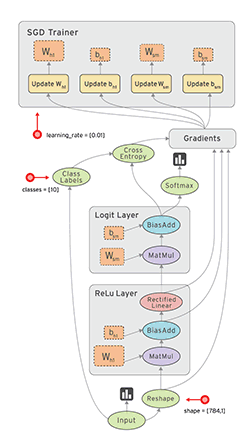# TensorFlow Tutorial : A Beginner’s Guide to TensorFlow (Part -1)

Original article can be found here (source): Deep Learning on Medium# TensorFlow Tutorial : A Beginner’s Guide to TensorFlow (Part -1)

What is tensorflow?

• Open Source software library by Google.
• Originally created for tasks with numerical computation.
• c/c++ Backend.
• Based on data flow graphs.
• Main application : Deep Nueral Network.

Why tensorflow ?

• Python and c++ API ( python API is more complete and generally easier to use. )
• It has faster compilation time in comparison to alternative Deep Learning libraries.
• It supports CPUs, GPUs, and even distributed processing in a cluster. It is a very important feature as you can train a neural network using CPU and multiple GPUs, which makes the models very efficient on large-scale systems.

What is a data flow graph?

TensorFlow’s structure is based on the execution of a data flow graph.

We create graph with a graphs with a following computational units:

• Nodes : It represent a mathematical operation.
• Edges : It represent the multi-dimensional arrays, known as a tensors.

we can easily visualize different parts of the graph, which is not an option while using other Python libraries such as Numpy or SciKit. The standard usage is to build a graph first and then execute it in a session.

What is a meaning of Tensor?

• Tensor is a multidimensional array. It can be zero dimensional, such as scalar values, one dimensional as a line or vector, or 2-dimensional, such as a Matrix, and so on.for example. But as you know, images have colors, and to add information about the colors, we need another dimension, and that’s when a 3-dimensional tensor becomes particularly helpful. Now, let’s take a look at a dataflow graph and see how tensors and operations build the graph. As mentioned before, in a dataflow graph, the nodes are called operations, which represent units of computation. The edges are tensors which represent the data consumed or produced by an operation.
• TensorFlow programs use a data structure called tensor to represent all the data. Any type of data you plan to use for your model can be stored in Tensors. Simply put, a Tensor is a multi-dimensional array (0-D tensor: scalar, 1-D tensor: vector, 2-D tensor: matrix, and so on). Hence, TensorFlow is simply referring to the flow of the Tensors in the computational graph.

GRAPH

The biggest idea about Tensorflow is that all the numerical computations are expressed as a computational graph. In other words, the backbone of any Tensorflow program is a Graph. Anything that happens in your model is represented by the computational graph. Now, let’s take a look at a dataflow graph and see how tensors and operations build the graph. As mentioned before, in a dataflow graph, the nodes are called operations, which represent units of computation. The edges are tensors which represent the data consumed or produced by an operation.

In this graph, Feature matrix is a placeholder. Placeholders can be seen as “holes” in your model — “holes” through which you can pass the data from outside of the graph. Placeholders allow us to create our operations in the graph, without needing the data. When we want to execute the graph, we have to feed placeholders with our input data. This is why we need to initialize placeholders before using them.

Let’s look at another operation which builds the variables for our program.
In this graph, Weight Matrix is a variable. TensorFlow variables, are used to share and persist some values, that are manipulated by the program.

Please notice that when you define a place- holder or variable, TensorFlow adds an operation to your graph. In our graph, “Weight matrix” and “Feature
matrix” should be multiplied using a multiplication operation. After that, Add operation is called, which adds the result of the previous operation with bias term. The output of each operation is a tensor. The resulting tensors of each operation crosses the next one until the end where it’s possible to get the desired result. After adding all these operations in a graph, we can create a session to run the graph, and perform the computations.

Architecture of TensorFlow.

TensorFlow comes with an easy to use Python interface to build and execute your computational graphs. But what makes TensorFlow so popular today, is
its architecture. TensorFlow’s flexible architecture allows you to deploy computation on one or more CPUs, or GPUs, or on a desktop, server, or even
a mobile device. This means you build your program once, and then you can run it easily on different devices.

Why Deep Learning with TensorFlow?

So let’s briefly review the reasons why TensorFlow is well-suited for deep learning applications. First, TensorFlow has built-in support for
deep learning and neural networks, so it’s easy to assemble a net, assign parameters, and run the training process. Second, it also has a collection of simple, trainable mathematical functions that are useful for neural networks.
Finally, deep learning as a gradient-based machine learning algorithm will benefit from TensorFlow’s auto-differentiation and optimizers.

References